Home | ARTS | Accounting For Managers | Additional Illustrations-Marginal Costing

Accounting For Managers - Management Accounting-Marginal Costing

Posted On :  03.05.2018 07:37 am

From the following information, find out the amount of profit earned during the year, using marginal cost equation:

Illustration 16:

from the following information, find out the amount of profit earned during the year, using marginal cost equation:
Fixed Cost                                                                                       Rs.5,00,000

Variable Cost                                                                                   Rs.10 Per Unit

Selling Price                                                                                    Rs.15 Per Unit

Output Level                                                                                      1,50,000 Units
Solution: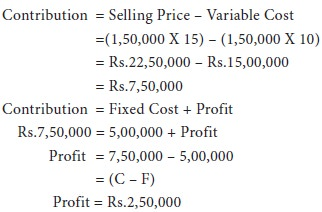Illustration 17:

Determine the amount of fixed costs from the following details, using the marginal cost equation.

 Sales Rs.2,40,000 Direct Materials Rs. 80,000 Direct Labour Rs. 50,000 Variable Overheads Rs. 20,000 Profit Rs. 50,000

Solution: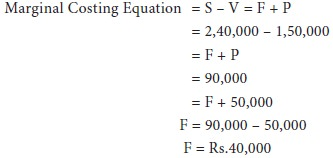Illustration 18:

Sales 10,000 Units @ Rs.25 Per Unit

Variable Cost Rs.15 Per Unit

Fixed Costs Rs.1,00,000

Find Out The Sales For Earning A Profit Of Rs.50,000
Solution:

Sales To Earn A Profit Of Rs.50,000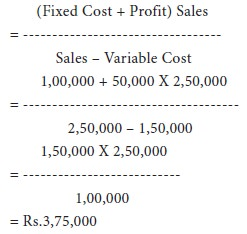Illustration 19:

The records of ram ltd., Which has three departments give the following figures: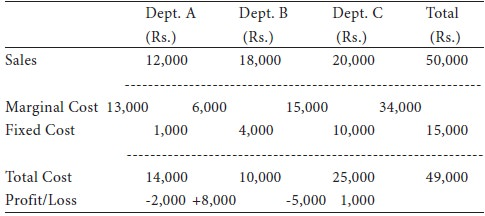The management wants to discontinue product c immediately as it gives the maximum loss. How would you advise the management?

Solution: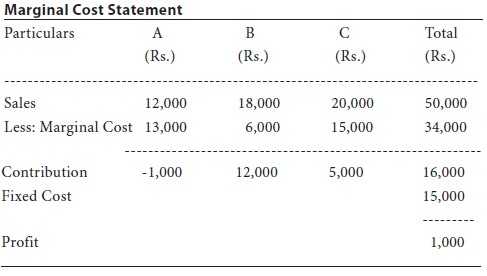Here department A gives negative contribution, and as such it can be given up. Department C gives a contribution of Rs.5,000. If department C is closed, then it may lead to further loss. Therefore, C should be continued.

Tags : Accounting For Managers - Management Accounting-Marginal Costing
Last 30 days 9 views